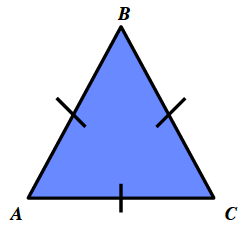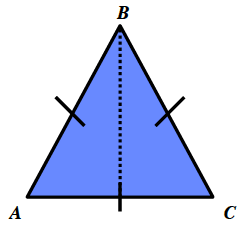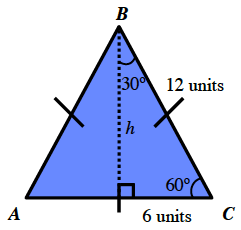### Home > CCG > Chapter Ch7 > Lesson 7.2.4 > Problem7-83

7-83.

Use Tromika’s method from problem 7‑78 to find the area of an equilateral triangle with side length $12$ units. Show all work. Homework Help ✎

Draw the triangle described.

Draw in the height of the triangle which bisects the base of the triangle.

Use the Pythagorean Theorem or the $30°-60°-90°$ triangle pattern to calculate the height of the triangle.

$h\approx10.39$ units

Use the height to find the area of the triangle.

$\left ( \frac{1}{2} \right )(12\text{ units})(10.39\text{ units})\approx 62.34 \text{ units}^{2}$